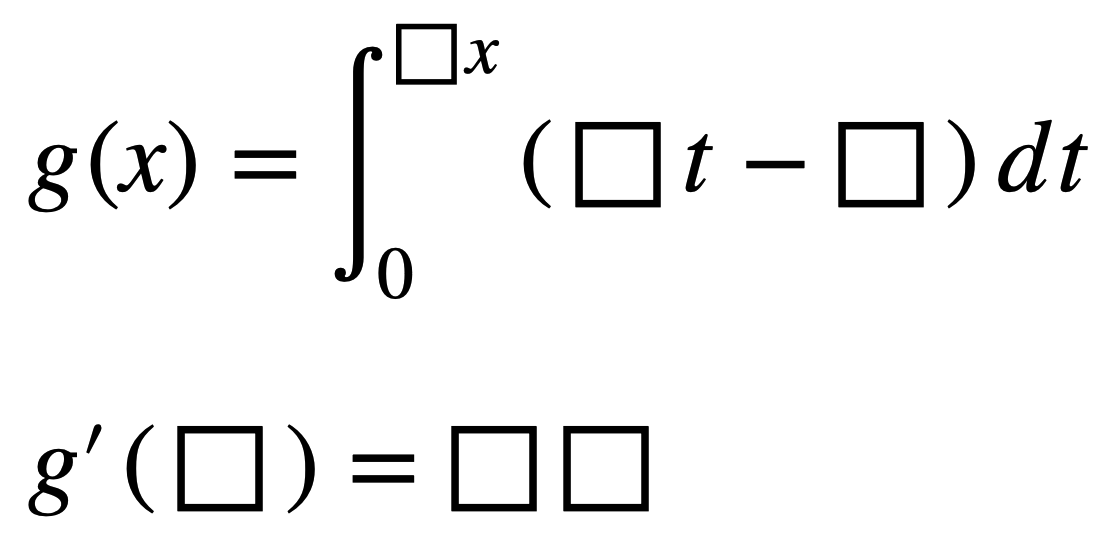# Fundamental Theorem Of Calculus

Directions: Using the digits 0 to 9 at most one time each, place a digit in each box to make a derivative as close to 100 as possible.### Hint

Try replacing the blanks – not the result of g'(_) – with a, b, c, d. Then compute the derivative algebraically.
g(x) = the integral of (at-b) with respect to t from 0 to Dx
g'(c) = _ _

g(x) = the integral of (at-b) with respect to t from 0 to Dx
g'(c) = _ _

(a, b, c, d, val) = ( 1 , 0 , 2 , 7 , 98 )
(a, b, c, d, val) = ( 2 , 0 , 1 , 7 , 98 )

If the minus sign in the integrand is changed to a plus sign, the solutions are
(a, b, c, d, val) = ( 1 , 0 , 2 , 7 , 98 )
(a, b, c, d, val) = ( 2 , 0 , 1 , 7 , 98 )
(a, b, c, d, val) = ( 4 , 1 , 6 , 2 , 98 )
(a, b, c, d, val) = ( 6 , 1 , 4 , 2 , 98 )

Source: Stephen Spinelli

## Limits

Directions: Using the digits 0 to 9 at most one time each, place a digit …

### One comment

1.